# Question 5.7 5.7 Multiply the local balance of linear momentum dyadically with the velocity to show that [a(uta) (PM...

Question 5.7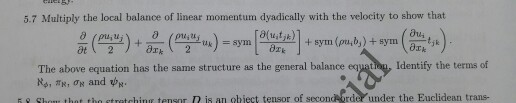5.7 Multiply the local balance of linear momentum dyadically with the velocity to show that [a(uta) (PMAW pu,u + pu,u, + sym (pu,b,) + sym =sym 2 at 2 The above equation has the same structure as the general balance equation, Identify the terms of No, , OR and ER Shom that tho etretching tensor D is an obiect tensor of secondorder under the Euclidean trans-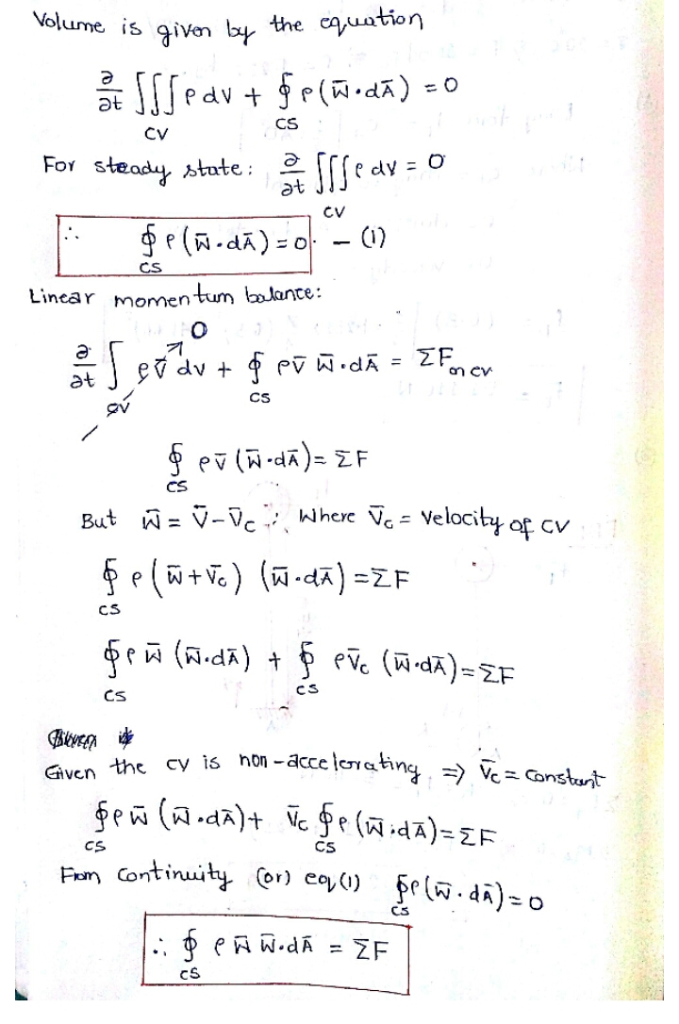##### Add Answer of: Question 5.7 5.7 Multiply the local balance of linear momentum dyadically with the velocity to show that [a(uta) (PM...
Similar Homework Help Questions
• ### A strange way of differential equation solving without know- ing the Fundamental Theorem of Calcu...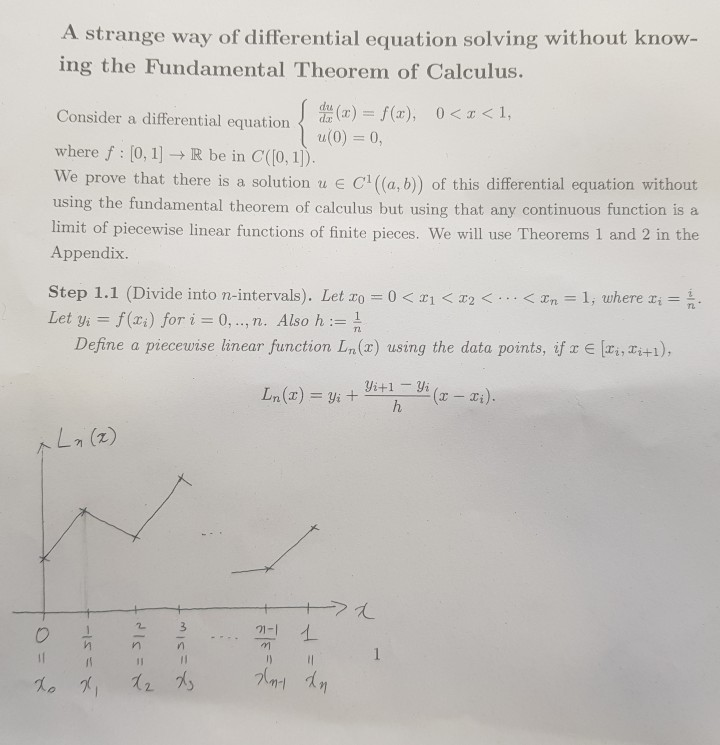problem 1, 2-1, 2-2, 3, 4 and f is nonnegative A strange way of differential equation solving without know- ing the Fundamental Theorem of Calculus. ! 忑(x) = f(x), 0 < x < 1, Consider a differential equation where f : [0, 1] → R be in C(0,1)) We prove that there is a solution u e C(a,b) of this differential equation without using the fundamental theorem of calculus but using that any continuous function is a limit of piecewise...

• ### In the decay 32P →32 S + e-find: a) the energy of the electron b) the energy of the core 32S c) the linear momentum...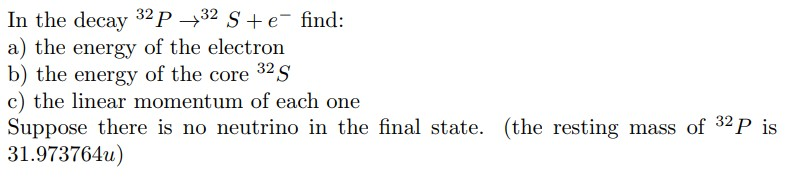In the decay 32P →32 S + e-find: a) the energy of the electron b) the energy of the core 32S c) the linear momentum of each one Suppose there is no neutrino in the final state. (the resting mass of 32P is 31.973764u) In the decay 32P →32 S + e-find: a) the energy of the electron b) the energy of the core 32S c) the linear momentum of each one Suppose there is no neutrino in the final...

• ### can you please post the answer thanks FE yIn] x[n] -2 3. Given the causal LTI system with signal flow graph as shown (a) Determine the system function H(z) (5) (b) Determine the minimum multiply l...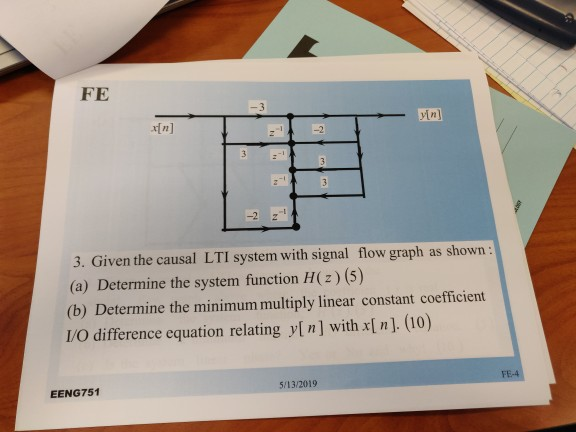can you please post the answer thanks FE yIn] x[n] -2 3. Given the causal LTI system with signal flow graph as shown (a) Determine the system function H(z) (5) (b) Determine the minimum multiply linear constant coefficient /O difference equation relating y[n] with x[n]. (10) EENG751 5/13/2019 FE yIn] x[n] -2 3. Given the causal LTI system with signal flow graph as shown (a) Determine the system function H(z) (5) (b) Determine the minimum multiply linear constant coefficient /O...

• ### 2. Let u(z,t) be a differentiable function on R x [0, 0o). a) Show that the directional derivativ...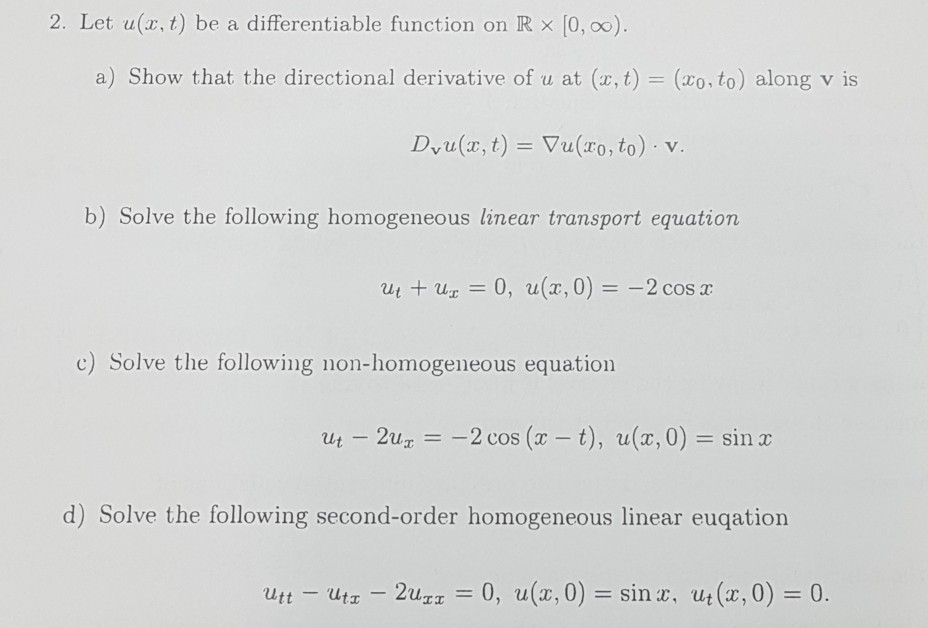2. Let u(z,t) be a differentiable function on R x [0, 0o). a) Show that the directional derivative of u at (x, t) = (zo, to) along v is Dvu(x, t) = ▽u(ro, to) , v b) Solve the following homogeneous linear transport equation ul + uz = 0, u(x,0) =-2 cosx c) Solve the following non-homogeneous equation ut-2uz--2 cos (x-t), u(x, 0) = sin x d) Solve the following second-order homogeneous linear euqation u(z,0) = sin x, ut (z,...

• ### 2.The angular momentum is L = p a) What is the representation of the angular momentum operator b)Use the polar coordinates to compute L o)Show that the eigenfunction forp) m(p),where mis integer....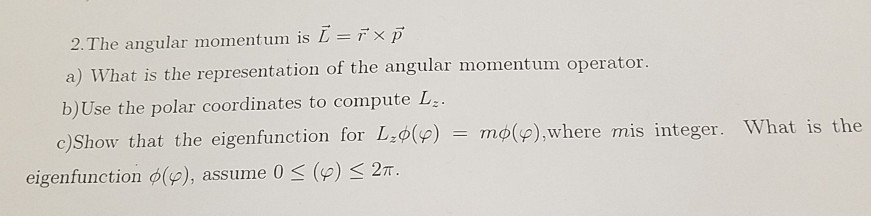2.The angular momentum is L = p a) What is the representation of the angular momentum operator b)Use the polar coordinates to compute L o)Show that the eigenfunction forp) m(p),where mis integer. What is the Τηφ(p),where What mis integer. is the eigenfunction φ(p), assume 0 (p) 2π 2.The angular momentum is L = p a) What is the representation of the angular momentum operator b)Use the polar coordinates to compute L o)Show that the eigenfunction forp) m(p),where mis integer. What...

• ### 2. a) Balance the equation below and use it to calculate the moles of Fe formed from 0.50 moles of Fe,O3: Fe2O3(...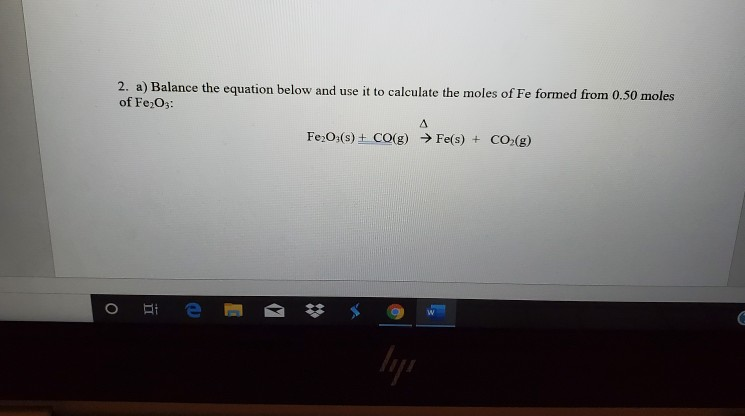2. a) Balance the equation below and use it to calculate the moles of Fe formed from 0.50 moles of Fe,O3: Fe2O3(s) + CO(g) → Fe(s) + CO2(g) 3. Balance the equation below then calculate the mass of ZnCl2 by obtained by reacting 10.0 g of zinc metal with an excess (more than is required) of hydrochloric acid Zn(s) + HCl(aq) → ZnCl2(aq) + H2(g) Morse backs pause

• ### QUESTION 2 Given that the results of a linear shrinkage test were Length before drying 160 mm Length after drying-14...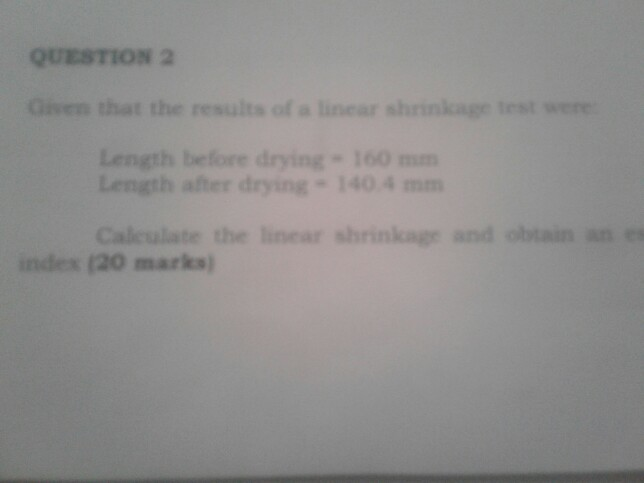QUESTION 2 Given that the results of a linear shrinkage test were Length before drying 160 mm Length after drying-140 4 mm Caleulate the linear shrinkagr and obtain an es index (20 marks) Show transcribed image text

• ### Linear Algebra Question: Forgot to include the vaules of u, v and w.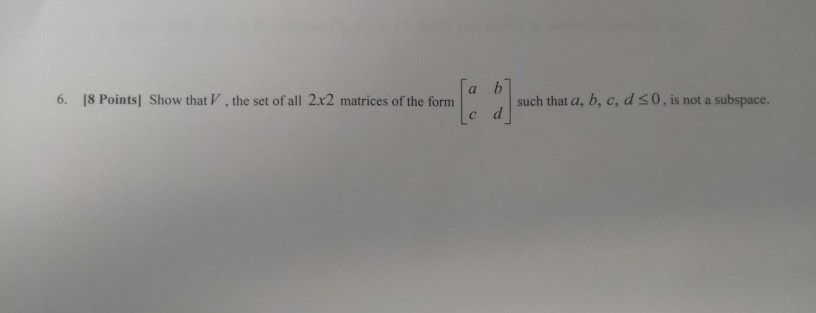Linear Algebra Question: Forgot to include the vaules of u, v and w. :]suchthata,b,c,dso 6. 18 Points] Show that V, the set of all 2x2 matrices of the form such that a, b, c, d s0, is not a subspace. s. I5 Points each] Let ü = (2,-6, 2), v = (0, 4,-2), and w-(2, 2,-4) a. Find a vector that is orthogonal to both v and w using the cross product. b. Find the area of the parallelogram in...

• ### Fr the falling fm . Lerive anl vcloci Pey o 42) assumin 5 usinte equatienmtion (6.5-3), niam it...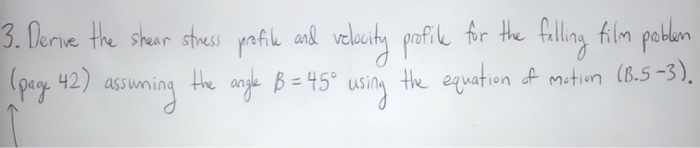fr the falling fm . Lerive anl vcloci Pey o 42) assumin 5 usinte equatienmtion (6.5-3), niam ity, average velocity, or force on solid surfaces. tion appear, and In the integrations mentioned above, several constants of integration a the velocit stress at the boundaries of the system. The most commonly used boundae are as follows: using "boundary conditions"-that is, statements about a. At solid-fluid interfaces the fluid velocity equals the velocity with which surface is moving: this statement is applied...

• ### 1) Linearity: In order for a system to be linear it must satisfy the following equation: In other...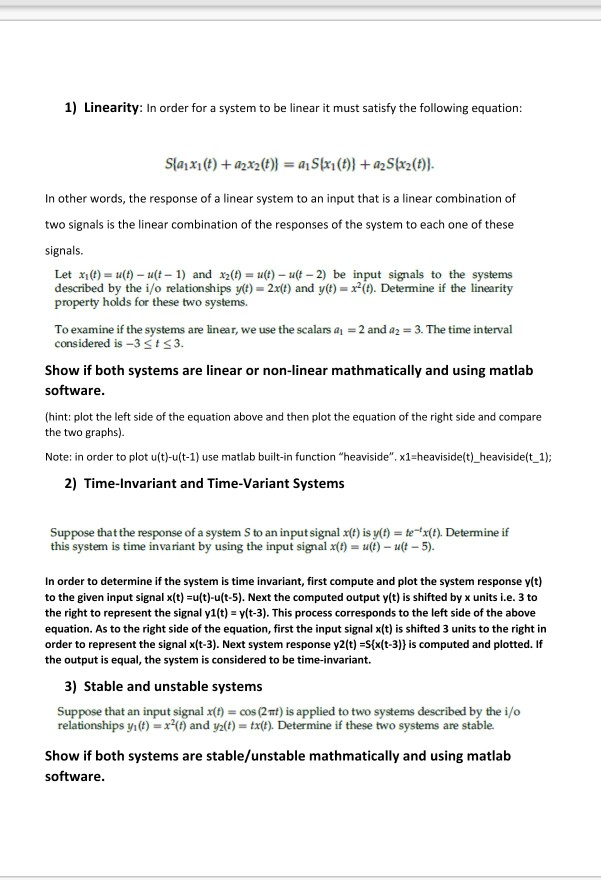need solution and code for this signal and system problem 1) Linearity: In order for a system to be linear it must satisfy the following equation: In other words, the response of a linear system to an input that is a linear combination of two signals is the linear combination of the responses of the system to each one of these signals. Let xi)- u(t) -u(t-1) and x2t) u- u(t-2) be input signals to the systems described by the i/o...

Need Online Homework Help?